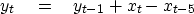Next: Smoothing with a triangle Up: SMOOTHING WITH BOX AND Previous: SMOOTHING WITH BOX AND

## Smoothing with a rectangle

The inverse of any polynomial reverberates forever, although it might drop off fast enough for any practical need. On the other hand, a rational filter can suddenly drop to zero and stay there. Let us look at a popular rational filter, the rectangle or box car'':(10)
The filter (10) gives a moving average under a rectangular window. This is a basic smoothing filter. A clever way to apply it is to move the rectangle by adding a new value at one end while dropping an old value from the other end. This approach is formalized by the polynomial division algorithm, which can be simplified because so many coefficients are either one or zero. To find the recursion associated with Y(Z)= X(Z)(1-Z5)/(1-Z), we identify the coefficient of Zt in (1-Z)Y(Z)= X(Z)(1-Z5). The result is(11)
This approach boils down to the program boxconv(), which is so fast it is almost free!
subroutine boxconv( nb, nx, xx, yy)
# inputs:       nx,  xx(i), i=1,nx      the data
#               nb                      the box length
# output:       yy(i),i=1,nx+nb-1       smoothed data
integer nx, ny, nb, i
real xx(nx), yy(1)
temporary real bb(nx+nb)
if( nb < 1 || nb > nx)  call erexit('boxconv')  # "||" means .OR.
ny = nx+nb-1
do i= 1, ny
bb(i) = 0.
bb(1) = xx(1)
do i= 2, nx
bb(i) = bb(i-1) + xx(i)         # make B(Z) = X(Z)/(1-Z)
do i= nx+1, ny
bb(i) = bb(i-1)
do i= 1, nb
yy(i) = bb(i)
do i= nb+1, ny
yy(i) = bb(i) - bb(i-nb)        # make Y(Z) = B(Z)*(1-Z**nb)
do i= 1, ny
yy(i) = yy(i) / nb
return; end

Its last line scales the output by dividing by the rectangle length. With this scaling, the zero-frequency component of the input is unchanged, while other frequencies are suppressed.

Let us examine the pole and zero locations in equation (10). The denominator vanishes at Z=1, so the filter has a pole at zero frequency. Smoothing something is like boosting frequencies near the zero frequency. The numerator vanishes at the five roots of unity, i.e.,.These five locations are uniformly spaced around the unit circle. Any sinusoid at exactly one of these frequencies is exactly destroyed by this filter, because such a sinusoid has an integer number of wavelengths under the boxcar. An exception is the zero frequency, where the root at Z=1 is canceled by a pole at the same location. This cancellation is the reason the right-hand side ends at the fourth power--there is no infinite series of higher powers.Next: Smoothing with a triangle Up: SMOOTHING WITH BOX AND Previous: SMOOTHING WITH BOX AND
Stanford Exploration Project
10/21/1998